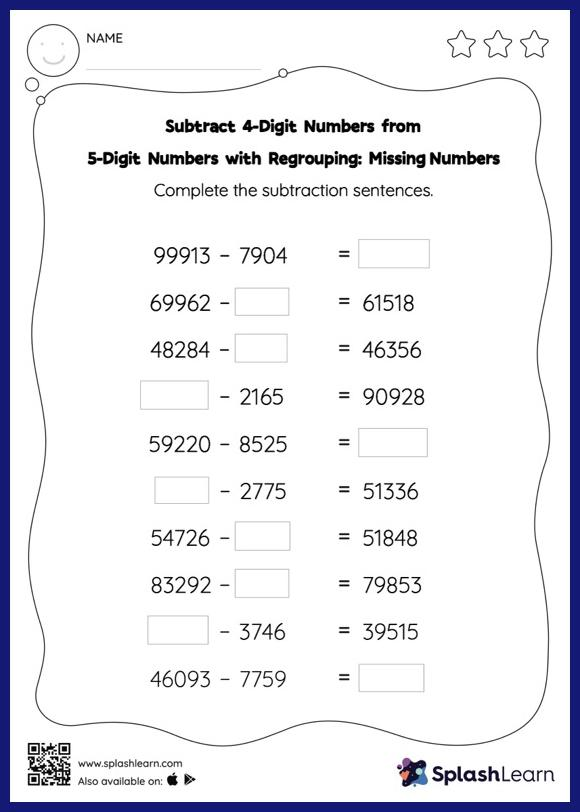# Subtract 4-Digit Numbers from 5-Digit Numbers with Regrouping: Missing Numbers Worksheet

Home > Subtract 4-Digit Numbers from 5-Digit Numbers with Regrouping: Missing NumbersTo find the missing number on this worksheet, students can utilize the count back strategy or the connection between addition and subtraction. In subtract 4-digit numbers from 5-digit numbers with regrouping worksheet, they must additionally regroup numbers to reach the solution. As the worksheet uses a horizontal format, it allows for more creativity when it comes to how the student solves the problem. In contrast, in the vertical format, students usually employ the standard method to solve.